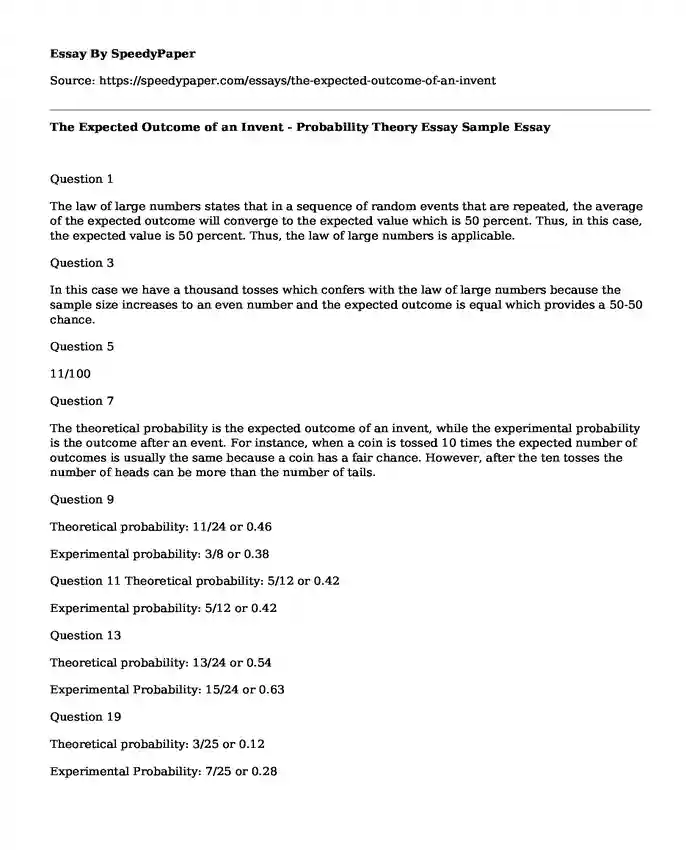# The Expected Outcome of an Invent - Probability Theory Essay Sample

Published: 2017-11-16Type of paper: Essay Categories: Mathematics Pages: 1 Wordcount: 217 words
143 views

Question 1

Is your time best spent reading someone else’s essay? Get a 100% original essay FROM A CERTIFIED WRITER!

The law of large numbers states that in a sequence of random events that are repeated, the average of the expected outcome will converge to the expected value which is 50 percent. Thus, in this case, the expected value is 50 percent. Thus, the law of large numbers is applicable.

Question 3

In this case we have a thousand tosses which confers with the law of large numbers because the sample size increases to an even number and the expected outcome is equal which provides a 50-50 chance.

Question 5

11/100

Question 7

The theoretical probability is the expected outcome of an invent, while the experimental probability is the outcome after an event. For instance, when a coin is tossed 10 times the expected number of outcomes is usually the same because a coin has a fair chance. However, after the ten tosses the number of heads can be more than the number of tails.

Question 9

Theoretical probability: 11/24 or 0.46

Experimental probability: 3/8 or 0.38

Question 11 Theoretical probability: 5/12 or 0.42

Experimental probability: 5/12 or 0.42

Question 13

Theoretical probability: 13/24 or 0.54

Experimental Probability: 15/24 or 0.63

Question 19

Theoretical probability: 3/25 or 0.12

Experimental Probability: 7/25 or 0.28

Question 20

Theoretical probability: 3/25 or 0.12

Experimental probability: 13/50 0.26

The Expected Outcome of an Invent - Probability Theory Essay Sample. (2017, Nov 16). Retrieved from https://speedypaper.com/essays/the-expected-outcome-of-an-invent

Request Removal

If you are the original author of this essay and no longer wish to have it published on the SpeedyPaper website, please click below to request its removal:

Liked this essay sample but need an original one?

Hire a professional with VAST experience!